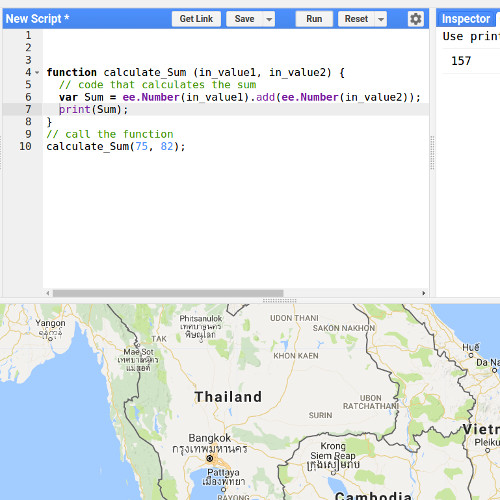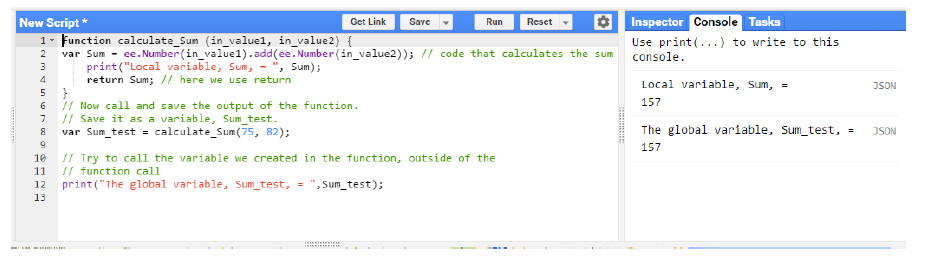# Writing functions in the Google Earth Engine

This is really helpful to create more efficient code!

This introductory training is part of the official training materials prepared by the United States Department of Agriculture.

The Code Editor offers access to the full power of Earth Engine; however, a basic understanding of the fundamentals of coding and JavaScript is required. In this exercise, you will continue to learn about JavaScript syntax and some new Earth Engine spatial data concepts. You will build what you learned in exercise two about image objects; however you will now turn your focus to working with collections of images, or stacks of similar image objects. In this exercise, you will focus on basic concepts and methods associated with image collections in Earth Engine. This is an introduction to get users writing some simple JavaScript scripts.

# Functions Primer

As you develop your code, it can start getting quite long. To keep it organized and create more efficient code, you can re-use pieces you’ve built by taking advantage of functions. You simply break apart your code into separate pieces, wrap these pieces up, and give them a name that we can call to apply later when needed. Functions are essentially smaller re-useable modular pieces of code. For example, we might have a function to get the data set(s) of interest, one to analyze them, and finally another to export them. We can break the code up into these three parts – each wrapped up as a function.

Functions are also highly useful when you have a series of statements that you would like to repeat many times in the code. For example, perhaps we wanted to calculate the mean of our data or the normalized difference vegetation index (NDVI) of a series of rasters, we could create a function that we could call each time we wanted to execute either of these in our code, rather than have to re-write those pieces each time.

Structure

Here’s how functions are built:

Code: You take the code you want to enclose (one statement or hundreds of lines) and wrap it in curly braces to note where the function starts and ends. This goes where the green comment lines are in the code block example below.

function: Write the JavaScript word function, all in lower case, before the first curly brace.

name_of_function: The word function is followed by the name we would like to call our function. We can name our function anything we would like, the same rules and style suggestions of how to name variables apply to naming functions (can’t start with a number, should be descriptive of what it does, etc).

Input parameters: In between our function name and our first curly brace we need to add parenthesis. These are filled with the set of input parameters that the user passes to the function, if there are none it can be left empty. An input parameter is a piece of information (it can be stored in a variable) that is passed into the function when it is called. If you have many parameters that you would like to include simply separate them with commas. Using the example of creating a function to calculate the NDVI of an image, we would pass the function the image on which we would like to apply the NDVI function.

Call the function: Once you’ve created a function, it doesn’t execute unless you call it. To use the function with in your code you write the name of the function, followed by parenthesis, with the required input parameters inside the parenthesis, and end the statement with a semicolon.

```function name_of_function (parameter_1, parameter_2) {
// code to execute
// more code to execute
// …
}

// call the function
name_of_function(input_1, input_2);
```

This is the basic structure of a function. If it sounds complicated, don’t worry, it will all make sense when you write one yourself. Below you’re going to practice by making a simple calculate_sum function.

Note: Common convention declares and specifies (all) the functions first, then calls them later in the script. It’s not required, but it makes for code that is easier to read.

Create a function to calculate the sum of numbers

1. Open a new space to begin working in by clicking the down arrow next to Reset. Then select Clear Script.

2. Assess the code below as an example of a function that calculates the sum of two values.

```function calculate_Sum (in_value1, in_value2) {
var Sum = ee.Number(in_value1).add(ee.Number(in_value2)); // code that calculates the sum
print(Sum);
}
// call the function
calculate_Sum(75, 82);
```
Note: So far we have looked at code that executes sequentially. Functions are a little different in that they can be defined anywhere in the code, but only execute when they are called. The example below is the same as the example above. Many programmers will group all of their functions at the beginning of a script, but those functions only execute when they are called later in the code.

3. Now above the area where you have created your function, type in two different values to sum and execute the code. An example statement is included below.

```calculate_Sum(99, 106);
// copy the call statement (above), below the creation of the function
function calculate_Sum (in_value1, in_value2) {
var Sum = ee.Number(in_value1).add(ee.Number(in_value2)); // code that calculates the sum
print(Sum);
}
```
Note: If the call to use these functions looks familiar, that is good! We have been using built-in JavaScript functions (or methods) in this exercise already. An example is print().

4. Once a function has been created, you can call the function as many times as you like. Let’s call it again with new parameters. This time add two numbers of your own choice.

```calculate_Sum(790, 1.555);
calculate_Sum(133, 765);
```

Global and Local Variables

1. What if we want to save the sum that we just calculated as a variable to use later on in our code, can we do that? We create and define a variable called Sum in the function. Try to print that variable after calling the function to access the output value. Type in the following below the creation of the function and execute the script.

```&amp;nbsp;// call the function
calculate_Sum(75, 82);
print(Sum);
```

2. That didn’t work. Try to save it in a different format. Delete the code that you added in the last step. Examine the code below. Add it to the script and Run the code.

```//call the function and save it as a variable called Sum_test
var Sum_test = calculate_Sum(75, 82);
// Try to call the variable we created in the function,
// outside of the function call
print(Sum_test);
```

What happened? We didn’t get an error message, however saving it as a variable didn’t work either. I got a message that a property cannot be read.

3. So far we haven’t had any success accessing the function output. This is because the Sum variable is a local variable, a variable that only exists and is relevant inside the function itself. In JavaScript anytime var is used inside a function, the resulting variable is a local variable that is only accessible within the function.

What’s a local variable? What’s a global variable?
A local variable is a variable that is declared inside of a function. Unlike a global variable, a local variable may only be used inside of the function it is declared in.A variable defined outside a function is a “global variable” and is visible everywhere, including inside functions. So far all you have learned about is global variables, though we haven’t been calling them global variables. A global variable is a variable that is declared outside of any functions. These variables can be used anytime in the code, and can also be used inside functions.

Use “return” to save the result of function operations as a variable

So how do we pass the local result of a function to a global variable? JavaScript uses a “return” statement to return a local value back to the main program. By declaring a global variable and setting it equal to the function call we assign the result of the function to a variable that can be used elsewhere in the script.

1. Examine the lines below. Insert them into your code editor script and click Run.

```function calculate_Sum (in_value1, in_value2) {
var Sum = ee.Number(in_value1).add(ee.Number(in_value2)); // code that calculates the sum
print(&quot;Local variable, Sum, = &quot;, Sum);
return Sum; // here we use return
}
// Now call and save the output of the function.
// Save it as a variable, Sum_test.
var Sum_test = calculate_Sum(75, 82);

// Try to call the variable we created in the function, outside of the
// function call
print(&quot;The global variable, Sum_test, = &quot;,Sum_test);
```

2. What happens? Now that appears to work! I see the value of 157 printed twice in my console:
i. Once as a local variable as the print output from running the function.
ii. The second time from calling the print function on my newly created global variable, Sum_test.1.Alexis Wasson says:

Is there any way you could provide a link to the USDA training materials you used to create this article?

1.thisearthsite says:

yes. Find the materials here: http://tinyurl.com/y4mo3nhk

2.Bopoto Bongo Pastor Letwyn says:

Am new in Google Earth Engine. can i have some tutorials on flood mapping using SAR Sentinel 1C band

3.Ernest G says:

Thank you very much, such valuable information.